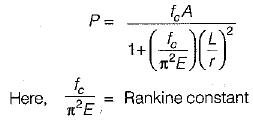Courses

# Test: Theory of Columns - 2

## 6 Questions MCQ Test Solid Mechanics | Test: Theory of Columns - 2

Description
This mock test of Test: Theory of Columns - 2 for Civil Engineering (CE) helps you for every Civil Engineering (CE) entrance exam. This contains 6 Multiple Choice Questions for Civil Engineering (CE) Test: Theory of Columns - 2 (mcq) to study with solutions a complete question bank. The solved questions answers in this Test: Theory of Columns - 2 quiz give you a good mix of easy questions and tough questions. Civil Engineering (CE) students definitely take this Test: Theory of Columns - 2 exercise for a better result in the exam. You can find other Test: Theory of Columns - 2 extra questions, long questions & short questions for Civil Engineering (CE) on EduRev as well by searching above.
QUESTION: 1

### The rectangular column shown in the figure below carries a load P having eccentricities ‘ex’ and ' ey ’ along the x-axis and y-axis respectively. The stress at any point (*, y) is given by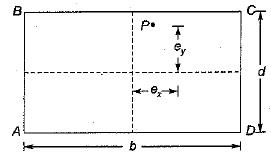Solution:

Stress at any point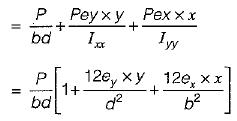QUESTION: 2

### Euler’s formula for a mild steel long column hinged  at both end is not valid for slenderness ratio

Solution:

According to Euler's theory,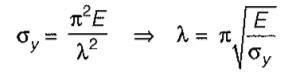For a mild steel coumn,
σy = 300 N/mm2 and
E = 2.1 x 105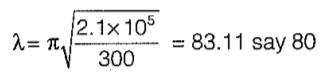Hence, when the slenderness ratio is less than this limit for mild steel columns , Euler’s formula will not be valid.

QUESTION: 3

### The buckling load for a column pinned at both ends is 10kN. If the ends are fixed, the buckling load changes to

Solution: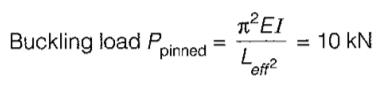For both ends pinned,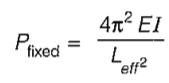= 4 x 10 = 40kN

QUESTION: 4

The radius of gyration of a rectangular section (depth d, width b) from a centroidal axis parallel to the width is

Solution: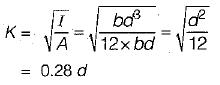QUESTION: 5

For no tension in column of diameter D, the eccentricity of the compressive load must be less than

Solution:

For no tension condition,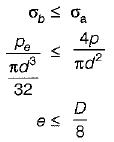QUESTION: 6

The Rankine constant in the formula for axial loads on column made of material of crushing strength fc and modulus of elasticity E is

Solution: